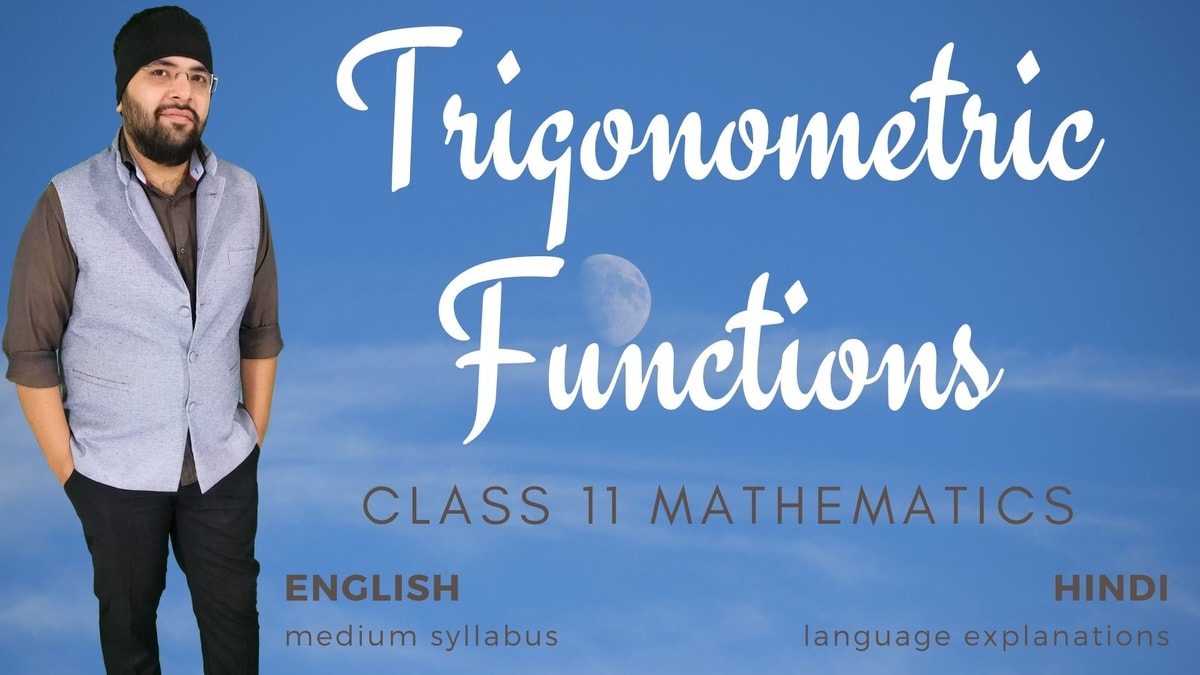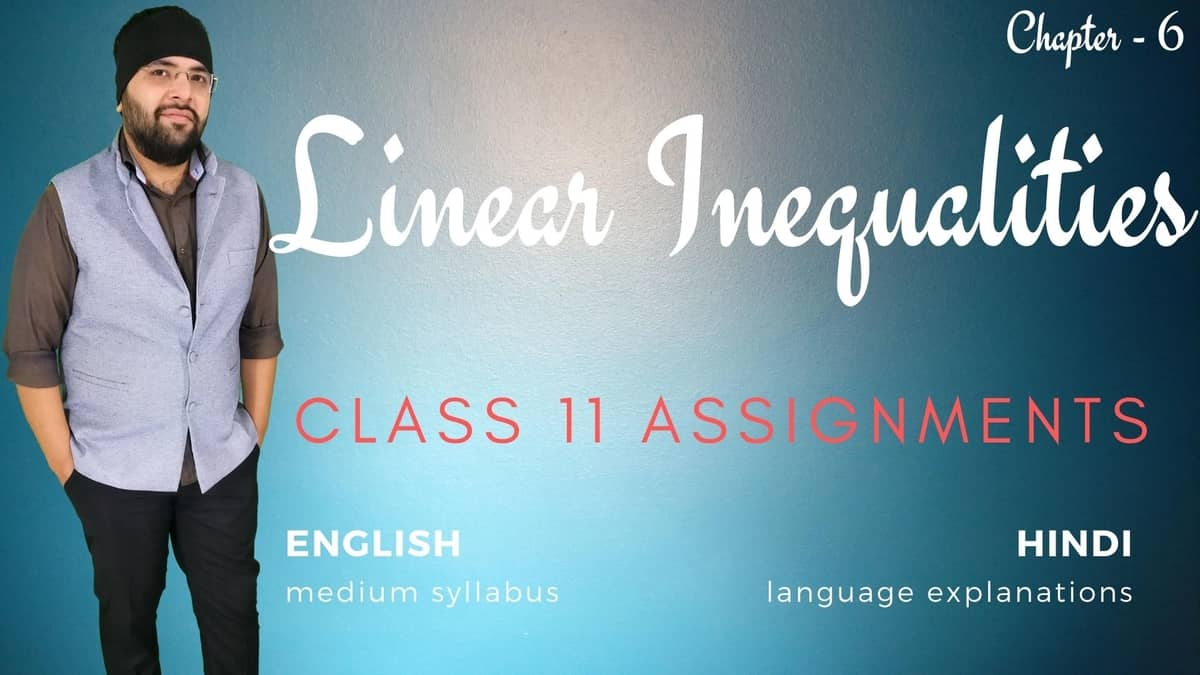## Select Chapter

### 1. Sets

Student### Sets Class 11 Maths

Sets and their representations. Empty set. Finite and Infinite sets. Equal sets. Subsets. Subsets of a set of real numbers especially intervals (with notations). Power set. Universal set. Venn diagrams. Union and Intersection of sets. Difference of sets. Complement of a set. Properties of Complement.

0% Complete
0/82 Steps

### 2. Relations and Functions

Members Only### Relations and Functions Class 11 Maths

Ordered pairs. Cartesian product of sets. Number of elements in the Cartesian product of two finite sets. Cartesian product of the set of reals with itself (upto R x R x R). Definition of relation, pictorial diagrams, domain, co-domain and range of a relation. Function as a special type of relation. Sum, difference, product and quotients of functions.

0% Complete
0/44 Steps

Members only### (Domain – Range) Relations and Functions Class 11 Maths

Pictorial representation of a function, domain, co-domain and range of a function. Real valued functions, domain and range of these functions, constant, identity, polynomial, rational, modulus, signum, exponential, logarithmic and greatest integer functions, with their graphs.

0% Complete
0/6 Steps

### 3. Trigonometric Functions

Members Only### Trigonometric Functions Class 11 Maths

Positive and negative angles.Measuring angles in radians and in degrees and conversion from one measure to another. Definition of trigonometric functions with the help of unit circle. Truth of the identity sin²x + cos²x = 1, for all x. Signs of trigonometric functions. Domain and range of trigonometric functions and their graphs.Expressing sin (x±y) and cos (x±y) in terms of sinx, siny, cosx & cosy and their simple applications. Deducing identities.

0% Complete
0/14 Steps

### 5. Complex Numbers and Quadratic Equations

Members Only### Complex Numbers Class 11 Maths

Need for complex numbers, especially $$\sqrt{-1}$$, to be motivated by inability to solve some of the quardratic equations. Algebraic properties of complex numbers.Argand plane and polar representation of complex numbers.Statement of Fundamental Theorem of Algebra, solution of quadratic equations (with real coefficients) in the complex number system.Square root of a complex number.

0% Complete
0/47 Steps

Members Only### Complex Numbers and Quadratic Equations Class 11 Assignments

This course is based on the Assignments by Ashish Kumar (Agam Sir). The assignments have questions from NCERT Exemplar, Board's Question Bank, R. D. Sharma etc., under the syllabus of Class 11 Mathematics.

0% Complete
0/31 Steps

### 6. Linear Inequalities

Members Only### Linear Inequalities Class 11 Maths

Linear inequalities.Algebraic solutions of linear inequalities in one variable and their representation on the number line.Graphical solution of linear inequalities in two variables.Graphical method of finding a solution of system of linear inequalities in two variables.

0% Complete
0/61 Steps

12 Months Members Only### Linear Inequalities Assignments Class 11 Maths

This course is based on the Assignments by Ashish Kumar (Agam Sir). The assignments have questions from NCERT Exemplar, Board's Question Bank, R. D. Sharma etc., under the syllabus of Class 11 Mathematics.

0% Complete
0/25 Steps

### 9. Sequences and Series

Members Only### Sequences and Series Class 11 Maths

Syllabus: Sequence and Series. Arithmetic Progression (A. P.). Arithmetic Mean (A.M.) Geometric Progression (G.P.), general term of a G.P., sum of n terms of a G.P., infinite G.P. and its sum, geometric mean (G.M.), relation between A.M. and G.M.

0% Complete
0/119 Steps

### 10. Straight Lines

Members Only### Straight Lines Class 11 Maths

Syllabus: Brief recall of two dimensional geometry from earlier classes. Shifting of origin. Slope of a line and angle between two lines. Various forms of equations of a line: parallel to axis, point -slope form, slope-intercept form, two-point form, intercept form and normal form. General equation of a line.

0% Complete
0/111 Steps

### 16. Probability

Members Only### Probability Class 11 Maths

Random experiments; outcomes, sample spaces (set representation). Events; occurrence of events, ‘not’, ‘and’ and ‘or’ events, exhaustive events, mutually exclusive events, Probability of an event, probability of ‘not’, ‘and’ and ‘or’ events.

0% Complete
0/73 Steps